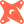Introducing PopSQL + dbt. The first SQL editor with built-in dbt support.Read more →

# How to Modify Arraysin PostgreSQL

## Overwriting an Array

The most basic way to modify an array column are to overwrite all values by assigning it a new array, or to specify an element to change.

Say you start off with these data:

``````player_number  |  round_scores
---------------+------------------
10002 | {91,92,93,95,99}
10001 | {95,92,96,97,98}
``````
``````-- overwrite all scores for a player
update player_scores set round_scores='{92,93,94,96,98}' where player_number=10002;

-- change only the score for the second round for player 10001
update player_scores set round_scores=94 where player_number=10001;``````

After performing these commands, the updated data are:

`````` player_number |   round_scores
---------------+------------------
10002 | {92,93,94,96,98}
10001 | {95,94,96,97,98}
``````

## Prepend and Append to an Array

PostgreSQL has functions that offer more ways to modify arrays. First, use `array_prepend()` and `array_append()` to add one element to the start and to the end of an array, respectively:

``````update player_scores set round_scores = array_prepend(0, round_scores);

update player_scores set round_scores = array_append(round_scores, 100);``````
`````` player_number |      round_scores
---------------+------------------------
10002 | {0,92,93,94,96,98,100}
10001 | {0,95,94,96,97,98,100}
``````

## Concatenate Multiple Arrays

To add an array to another array, use `array_cat()`.

``select array_cat('{1, 2}', ARRAY[3, 4]) as concatenated_arrays;``
`````` concatenated_arrays
---------------------
{1,2,3,4}
``````

The `||` operator can be used as a much simpler alternative to `array_prepend()`, `array_append()` and `array_cat()`:

``select 1 || array[2, 3, 4] as element_prepend;``
`````` element_prepend
-----------------
{1,2,3,4}
``````
``select array[1, 2, 3] || 4 as element_append;``
`````` element_append
----------------
{1,2,3,4}
``````
``select array['a', 'b', 'c'] || array['d', 'e', 'f'] as concat_array;``
`````` concat_array
---------------
{a,b,c,d,e,f}
``````

You can even add an array to a 2-dimensional array:

``select array[1, 2] || array[[4, 5],[6, 7]] as concat_2d_array;``
``````   concat_2d_array
---------------------
{{1,2},{4,5},{6,7}}
``````

## Removal from an Array

`array_remove()` removes all elements that matches the second parameter.

``select array_remove(round_scores,94) as removed_94 from player_scores;``
``````     removed_94
---------------------
{0,92,93,96,98,100}
{0,95,96,97,98,100}
``````

Note: `array_remove()` removes ALL occurences of the matching values

``select array_remove(ARRAY[1,2,3,2,5], 2) as removed_2s;``
`````` removed_2s
------------
{1,3,5}
``````

## Replace Elements in an Array

`array_replace()` replaces all elements that matches the second parameter with the third parameter.

``select array_replace(ARRAY[1,2,3,2,5], 2, 10) as two_becomes_ten;``
`````` two_becomes_ten
-----------------
{1,10,3,10,5}
``````

## Fill an Array

`array_fill()` takes three parametes. `array_fill()` returns an array pre-filled by the value of the first parameter. The second parameter defines how many elements to initialize with the given value. The optional third parameter defines the starting position of the array (defaults to 1).

``````insert into player_scores (player_number, round_scores) values
(10003, array_fill(95,array));``````

In other words, update the `player_scores` table with a new record for player_number 10003. All 5 of her scores will be 95.

``````insert into player_scores (player_number, round_scores) values
(10004, array_fill(90,array,array));``````

Similarly, you update the `player_scores` table with a new record for player_number 10004. However, his 5 scores of 90, will begin in position 3 in the array.

`````` player_number |      round_scores
---------------+------------------------
10003 | {95,95,95,95,95}
10004 | [3:7]={90,90,90,90,90}
``````

To shows that the scores array for player 10004 started with element position 3, simply query:

``````select
round_scores,
round_scores,
round_scores
from player_scores
where player_number in (10003, 10004);``````
`````` player_number | round_scores | round_scores | round_scores
---------------+--------------+--------------+--------------
10003 |           95 |           95 |           95
10004 |              |              |           90
``````

# Ready for a modern SQL editor?